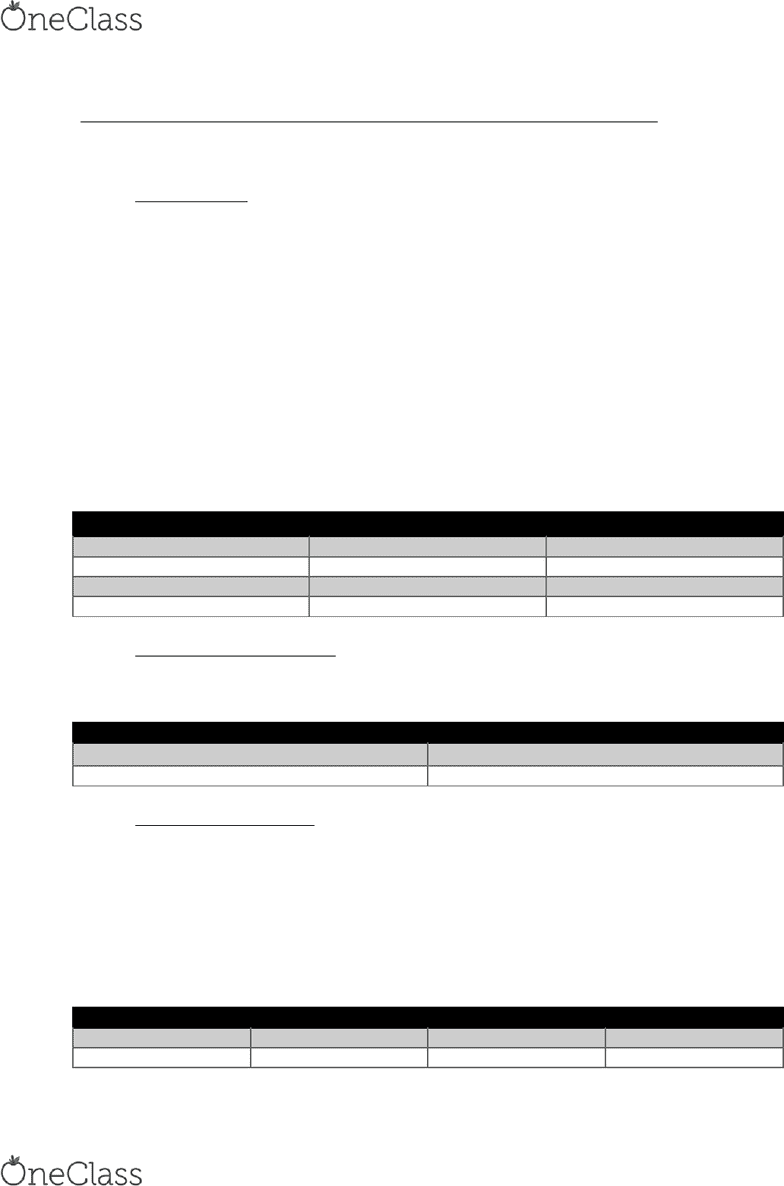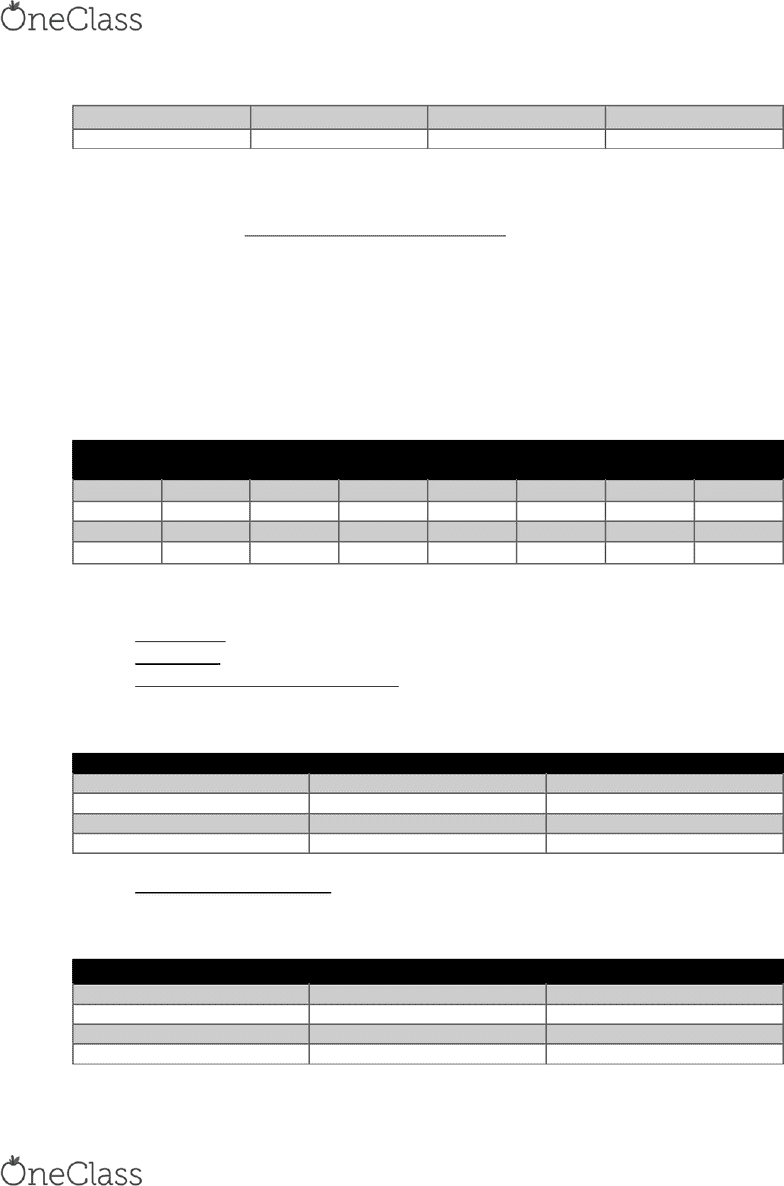Textbook Notes (270,000)
US (100,000)
UCSD (1,000)
PHIL (40)
Chapter 2

PHIL 10 Chapter Notes - Chapter 2: Counterexample, Truth Function

Department
Philosophy
Course Code
PHIL 10
Professor
Rick Grush
Chapter
2

This preview shows page 1. to view the full 5 pages of the document.PHIL 10 – Textbook Notes – Chapter 2: Truth Functions and Truth Tables
2.1 Truth functional operators and truth tables
Valid argument: all true premises and false conclusion not possible
2.1.1 Introduction
Operators are truth-functional
2.1.2 Functions
Truth functions take truth values (T/F) as inputs, produce truth values as outputs (T/F)
2.2 Truth functions: conjunction and negation
Only have 2 truth values
1. True and false/T and F
Conjunction truth table
X Y X · Y
TTT
TFF
FTF
FFF
Conjunction Truth Function: conjunction is true when both conjuncts are true, false if
either/both conjuncts are false
Negation truth table
X Y
T F
F T
Negation Truth Function: negation is true if negated statement false, false if negated
statement true
2.3 Truth tables for compound statements, and the point of formal systems
~ (A • B)
list all combinations of T/F for the statements in the first two columns
use conjunction truth function to fill out column 3
use negation truth function to fill out column 4
A B A • B ~ (A • B)
T T T F
T F F T
find more resources at oneclass.com
find more resources at oneclass.com

Unlock to view full version

Only page 1 are available for preview. Some parts have been intentionally blurred.F T F T
F F F T
to make a truth table:
1. determine atomic statements
2. find intermediate compound statements between atomic statements
intermediate compound statements: statements created by separating the
operators
3. make a table with a row for every combination of truth values of atomic
statements, column for every statement, put statements at top of each column,
starting with atomic in alphabetical order
~ (~~S • ~~L)
atomic statements = S and L
intermediate compound statements: ~S, ~~S, ~L, ~~L, ~~S • ~~L, ~ (~~S • ~~L)
L S ~L ~~L ~S ~~S ~~S •
~~L
~ (~~S •
~~L)
TTFTFTTF
TFFTTFFT
FTTFFTFT
FFTFTFFT
2.4 Truth functions: disjunction and conditional
Exclusive or: either P or Q but not both, excludes possibility of both disjuncts being true
Inclusive or: true when either or both disjuncts are true
Inclusive Disjunction Truth Function: disjunction is true if either or both disjuncts is true,
false if and only if both disjuncts false
Inclusive disjunction truth function
X Y X v Y
TTT
TFT
FTT
FFF
Conditional Truth Function: conditional false if it has combination of true antecedent and
false consequent, all other conditions conditional is true
Conditional truth table
X Y X ⸧ Y
TTT
TFF
FTT
FFT
find more resources at oneclass.com
find more resources at oneclass.com